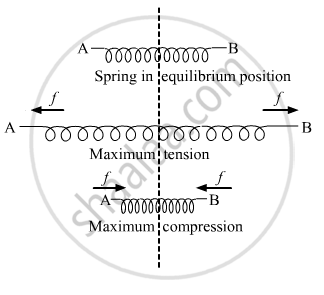Department of Pre-University Education, KarnatakaPUC Karnataka Science Class 11

# The Two Ends of a Spring Are Displaced Along the Length of the Spring. All Displacement Have Equal Magnitudes. - Physics

Short Note

The two ends of a spring are displaced along the length of the spring. All displacement have equal magnitudes. In which case or cases the tension or compression in the spring will have a maximum magnitude ?

(a) the right end is displaced towards right and the left end towards left
(b) both ends are displaced towards right
(c) both ends are displaced towards left
(d) the right end is displaced towards left and the left end towards right.

#### Solution

(a) the right end is displaced towards right and the left end towards left
(d) the right end is displaced towards left and the left end towards right.

When the right end is displaced towards the right and the left end towards the left, then this is the case of tension (expansion) and the spring will have maximum displacement.

When the right end is displaced towards the left and the left end towards the right, then this is the case of compression and ,again, the spring will have maximum displacement.Concept: Potential Energy of a Spring
Is there an error in this question or solution?

#### APPEARS IN

HC Verma Class 11, Class 12 Concepts of Physics Vol. 1
Chapter 4 The Forces
MCQ | Q 6 | Page 62
Share